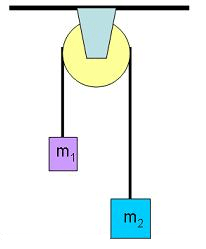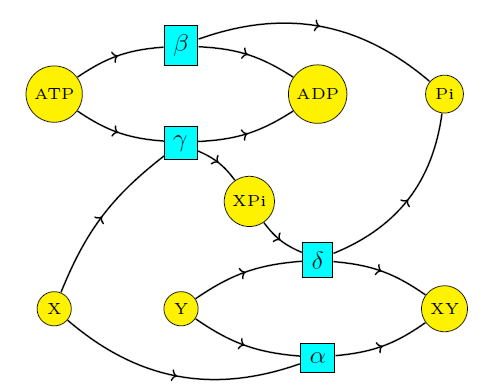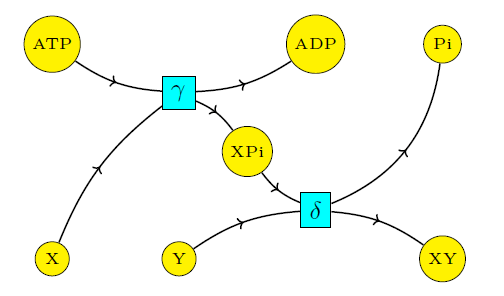## Coupling Through Emergent Conservation Laws (Part 6)

joint post with Jonathan Lorand, Blake Pollard, and Maru Sarazola

Now let’s think about emergent conservation laws!

When a heavy rock connected to a lighter one by a pulley falls down and pulls up the lighter one, you’re seeing an emergent conservation law:Here the height of the heavy rock plus the height of light one is a constant. That’s a conservation law! It forces some of the potential energy lost by one rock to be transferred to the other. But it’s not a fundamental conservation law, built into the fabric of physics. It’s an emergent law that holds only thanks to the clever design of the pulley. If the rope broke, this law would be broken too.

It’s not surprising that biology uses similar tricks. But let’s see exactly how it works. First let’s look at all four reactions we’ve been studying:$\begin{array}{cccc} \mathrm{X} + \mathrm{Y} & \mathrel{\substack{\alpha_{\rightarrow} \\\longleftrightarrow\\ \alpha_{\leftarrow}}} & \mathrm{XY} & \qquad (1) \\ \\ \mathrm{ATP} & \mathrel{\substack{\beta_{\rightarrow} \\\longleftrightarrow\\ \beta_{\leftarrow}}} & \mathrm{ADP} + \mathrm{P}_{\mathrm{i}} & \qquad (2) \\ \\ \mathrm{X} + \mathrm{ATP} & \mathrel{\substack{\gamma_{\rightarrow} \\\longleftrightarrow\\ \gamma_{\leftarrow}}} & \mathrm{ADP} + \mathrm{XP}_{\mathrm{i}} & \qquad (3) \\ \\ \mathrm{XP}_{\mathrm{i}} +\mathrm{Y} & \mathrel{\substack{\delta_{\rightarrow} \\\longleftrightarrow\\ \delta_{\leftarrow}}} & \mathrm{XY} + \mathrm{P}_{\mathrm{i}} & \qquad (4) \end{array}$

It’s easy to check that the rate equations for these reactions have the following conserved quantities, that is, quantities that are constant in time:

A)$[\mathrm{X}] + [\mathrm{XP}_{\mathrm{i}} ] + [\mathrm{XY}],$ due to the conservation of X.

B)$[\mathrm{Y}] + [\mathrm{XY}],$ due to the conservation of Y.

C)$3[\mathrm{ATP}] +[\mathrm{XP}_{\mathrm{i}} ] +[\mathrm{P}_{\mathrm{i}}] +2[\mathrm{ADP}],$ due to the conservation of phosphorus.

D)$[\mathrm{ATP}] + [\mathrm{ADP}],$ due to the conservation of adenosine.

Moreover, these quantities, and their linear combinations, are the only conserved quantities for reactions (1)–(4).

To see this, we use some standard ideas from reaction network theory. Consider the 7-dimensional space with orthonormal basis given by the species in our reaction network:$\mathrm{ATP}, \mathrm{ADP}, \mathrm{P}_{\mathrm{i}}, \mathrm{XP}_{\mathrm{i}}, \mathrm{X}, \mathrm{Y}, \mathrm{XY}$

We can think of complexes like$\mathrm{ADP} + \mathrm{P}_{\mathrm{i}}$ as vectors in this space. An arbitrary choice of the concentrations of all species also defines a vector in this space. Furthermore, any reaction involving these species defines a vector in this space, namely the sum of the products minus the sum of the reactants. This is called the reaction vector of this reaction. Reactions (1)–(4) give these reaction vectors:$\begin{array}{ccl} v_\alpha &=& \mathrm{XY} - \mathrm{X} - \mathrm{Y} \\ \\ v_\beta &= & \mathrm{P}_{\mathrm{i}} + \mathrm{ADP} - \mathrm{ATP} \\ \\ v_\gamma &=& \mathrm{XP}_{\mathrm{i}} + \mathrm{ADP} - \mathrm{ATP} - \mathrm{X} \\ \\ v_\delta &= & \mathrm{XY} + \mathrm{P}_{\mathrm{i}} - \mathrm{XP}_{\mathrm{i}} - \mathrm{Y} \end{array}$

Any change in concentrations caused by these reactions must lie in the stoichiometric subspace: that is, the space spanned by the reaction vectors. Since these vectors obey one nontrivial relation:$v_\alpha + v_\beta = v_\gamma + v_\delta$

the stochiometric subspace is 3-dimensional. Therefore, the space of conserved quantities must be 4-dimensional, since these specify the constraints on allowed changes in concentrations.

Now let’s compare the situation where ‘coupling’ occurs! For this we consider only reactions (3) and (4):Now the stoichiometric subspace is 2-dimensional, since$v_\gamma$ and$v_\delta$ are linearly independent. Thus, the space of conserved quantities becomes 5-dimensional! Indeed, we can find an additional conserved quantity:

E)$[\mathrm{Y} ] +[\mathrm{P}_{\mathrm{i}}]$

that is linearly independent from the four conserved quantities we had before. It does not derive from the conservation of a particular molecular component. In other words, conservation of this quantity is not a fundamental law of chemistry. Instead, it is an emergent conservation law, which holds thanks to the workings of the cell! It holds in situations where the rate constants of reactions catalyzed by the cell’s enzymes are so much larger than those of other reactions that we can ignore those other reactions.

And remember from last time: these are precisely the situations where we have coupling.

Indeed, the emergent conserved quantity E) precisely captures the phenomenon of coupling! The only way for ATP to form ADP + Pi without changing this quantity is for Y to be consumed in the same amount as Pi is created… thus forming the desired product XY.

Next time we’ll look at a more complicated example from biology: the urea cycle.

The paper:

• John Baez, Jonathan Lorand, Blake S. Pollard and Maru Sarazola,
Biochemical coupling through emergent conservation laws.

The blog series:

Part 1 – Introduction.

Part 2 – Review of reaction networks and equilibrium thermodynamics.

Part 3 – What is coupling?

Part 4 – Interactions.

Part 5 – Coupling in quasiequilibrium states.

Part 6 – Emergent conservation laws.

Part 7 – The urea cycle.

Part 8 – The citric acid cycle.

### 10 Responses to Coupling Through Emergent Conservation Laws (Part 6)

1.ayushkhaitan3437 says:

The potential energy lost by the heavy rock is not transferred completely to the light rock. For the same drop in height, the potential energy lost by the heavy rock will be greater than the potential energy gained by he lighter rock as their masses are different. The residual loss in potential energy is converted into kinetic energy of the rope, moving parts of the pulley, etc.

•John Baez says:

True! I’ll edit my post slightly.

2.A. Mani says:

The central idea can be generalized to applied logic and reasoning in ways that differ from the already existing ideas of emergence in AI.

•John Baez says:

How?

3.Peter Lichtner says:

If the reactions are coupled to diffusion and each species diffuses with its own diffusion coefficient then it would appear that the conservation laws are broken (maybe only slightly depending on how different the diffusion coefficients are).

4. […] Last time we examined ATP hydrolysis as a simple example of coupling through emergent conservation laws, but the phenomenon is more general. A slightly more complicated example is the urea cycle. The first metabolic cycle to be discovered, it is used by land-dwelling vertebrates to convert ammonia, which is highly toxic, to urea for excretion. Now we’ll find 11 conserved quantities in the urea cycle, including 7 emergent ones. […]

5. there must be 12 linearly independent conserved quantities. Some of these conservation laws are ‘fundamental’, at least by the standards of chemistry. All the species involved are made of 6 different atoms (carbon, hydrogen, oxygen, nitrogen, phosphorus and sulfur), and conservation of charge provides another fundamental conserved quantity, for a total of 7.

(In our example from last time we didn’t keep track of conservation of hydrogen and charge, because both$\mathrm{H}^+$ and$e^-$ ions are freely available in water… but we studied the citric acid cycle when we were younger, more energetic and less wise, so we kept careful track of hydrogen and charge, and made sure that all the reactions conserved these. So, we’ll have 7 fundamental conserved quantities.)

6.Scott Oswald says:

Any change in concentrations caused by these reactions must lie in the stoichiometric subspace: that is, the space spanned by the reaction vectors. Since these vectors obey one nontrivial relation:

[v_\alpha + v_\beta = v_\gamma + v_\delta]

the stochiometric subspace is 3-dimensional. Therefore, the space of conserved quantities must be 4-dimensional,

I see why the stochiometric subspace must be 3-dimensional, but I am not sure why the conserved quantities must be 4-dimensional. What determines the number of conserved quantities given a set of reaction vectors?

•John Baez says:

If you have$n$ species, the space$\mathbb{R}^n$ can be thought of as the space of possible concentrations of all species, but also any complex can be thought of as a vector in this space, and thus any change of complexes caused by a reaction can be thought of as a vector in this space, called the ‘reaction vector’. Given a specific reaction network, the collection of all reaction vectors span a subspace of$\mathbb{R}^n$ called the ‘stoichiometric subspace’. This describes the possible changes in concentration that could be caused by reactions in the reaction network.

On the other hand, any vector$u \in \mathbb{R}^n$ also determines a ‘quantity’ (possibly conserved, possibly not) that assigns a number to any given choice of concentrations. It does so using the dot product: if$c \in \mathbb{R}^n$ is a choice of concentrations, we get a number$u \cdot c.$

Thus, if$v$ is a vector in the stoichiometric subspace, which describes a possible change in concentrations,$u \cdot v$ will be the corresponding change in the quantity corresponding to$u.$

If$u$ is orthogonal to the whole stoichiometric subspace, this change will always be zero, so the quantity is conserved.

Thus, the orthogonal complement of the stoichiometric subspace is the space of conservation laws.

It follows that the dimension of the stoichiometric subspace plus the dimension of the space of conserved quantities equals$n.$

In other words, the maximum number of linearly independent reaction vectors plus the maximum number of linearly independent conservation laws is$n$.

In the example at hand this equation amounts to 3 + 4 = 7.

This stuff is part of reaction network theory. I think this is a pretty good, though long, introduction:

• Martin Feinberg, Lectures on Chemical Reaction Networks, 1979.

•Scott Oswald says:

Thanks for the explanation, that makes sense! Also thanks for the link to the lectures… definitely going to read through them sooner or later.

This site uses Akismet to reduce spam. Learn how your comment data is processed.